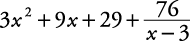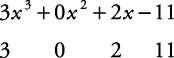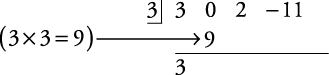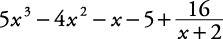## Synthetic Division

Synthetic division is a shortcut for polynomial division when the divisor is of the form xa. Only numeric coefficients of the dividend are used when dividing with synthetic division.

##### Example 1

Divide (2 x – 11 + 3 x 3) by ( x – 3).

First, this problem is done in the traditional manner. Then it is done by using the synthetic division method.

In the traditional manner,The answer is.

To do the problem using synthetic division, follow this procedure:

1. Write the polynomial being divided in descending order. Then write only its coefficients and constant, using 0 for any missing terms.2. Write the constant, a, of the divisor, xa, to the left. In this problem, a = 3 because you use the additive inverse of the constant. (Remember, the additive inverse of –3 is 3.)3. Bring down the first coefficient as shown.4. Multiply the first coefficient by the divisor, 3. Then write this product under the second coefficient.5. Add the second coefficient with the product and write the sum as shown.6. Continue this process of multiplying and adding until there is a sum for the last column.The numbers along the bottom row are the coefficients of the quotient with the powers of x in descending order. The last coefficient is the remainder. The first power is one less than the highest power of the polynomial that was being divided.

The division answer is.

##### Example 2

Divide (5 x 4 + 6 x 3 – 9 x 2 – 7 x + 6) by ( x + 2) using the synthetic method.

To put the divisor, x + 2, into the form xa, use the constant's negative. That means using x – (+2), so a = –2.The answer is.

Back to Top
A18ACD436D5A3997E3DA2573E3FD792A